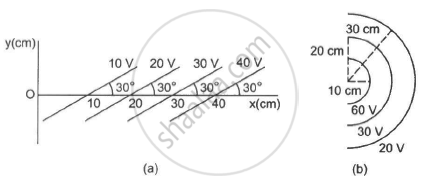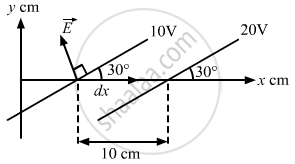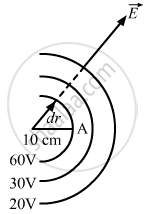Advertisement Remove all ads

# Some Equipotential Surface is Shown in the Figure. What Can You Say About the Magnitude and the Direction of the Electric Field? - Physics

Numerical

Some equipotential surface is shown in the figure. What can you say about the magnitude and the direction of the electric field?Advertisement Remove all ads

#### Solution

(a) The electric field is always perpendicular to the equipotential surface. (As shown in the figure)So, the angle between $\vec{E} \text{ and } \vec{dx}$ = $90^\circ+ 30^\circ$

Change in potential in the first and second equipotential surfaces, dV = 10 V
so,

$\vec{E} . \vec{dx} = -$ dV
$\Rightarrow \text{ Edx } \cos(90^\circ+ 30^\circ) = -$ dV
$\Rightarrow E(10 \times {10}^{- 2} )\cos120^\circ= - 10$
$\Rightarrow E = 200$ V/m
The electric field is making an angle of 120°  with the x axis.
(b) The electric field is always perpendicular to the equipotential surface.So, the angle between  $\vec{E}$ and $\vec{dr}$ = 0°
Potential at point A,
$V_A = \frac{1}{4\pi \epsilon_0}\frac{q}{r} =$60  V
$\Rightarrow \frac{q}{4\pi \epsilon_0} = 60 \times r$
$\Rightarrow \frac{q}{4\pi \epsilon_0} = 0 . 6$
So, electric field,

$E = \frac{q}{4\pi \epsilon_0} \times \frac{1}{r^2} = \frac{0 . 6}{r^2}$

The electric field is radially outward, decreasing with increasing distance.

Concept: Electric Field - Introduction of Electric Field
Is there an error in this question or solution?
Advertisement Remove all ads

#### APPEARS IN

HC Verma Class 11, Class 12 Concepts of Physics Vol. 2
Chapter 7 Electric Field and Potential
Q 62 | Page 123
Advertisement Remove all ads
Advertisement Remove all ads
Share
Notifications

View all notifications

Forgot password?